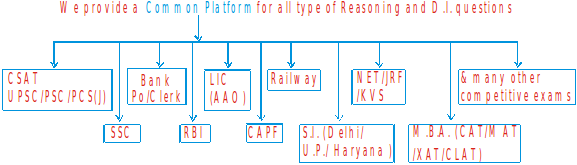First Batch – 9 : 15 A.M. to 11 : 00 A.M.

Second Batch – 11 : 15 A.M. to 1 : 00 PM

Third Batch – 4 : 15 P.M. to 6 : 00 P.M.

Fourth Batch – 6 : 30 P.M. to 8 : 00 P.M.

Special D.I. Batch 2 : 15 P.M. to 4 : 00 P.M.

Special D.I. Batch 8 : 00 P.M. to 9 : 15 P.M.

1. A. C.S.R (Common Sense Reasoning)

1. Problem Based on Alphabet Test

(a) Letter pair Question

(b) Alphabatical order of word

(c) Formation of word

2. Series (Letter & Number)

(a) alphabatical series

(b) alpha numeric series

3. Logical Sequence of words

4. Arrangement of words according to dictionary

5. alphabatical ranking based question

6. Letter pair based question

7. Mathematical Puzzles:

(a) Number Sequence Puzzle

(b) Mathematical Input Puzzle

(c) Arithmetical Logical & Mathematical operation

(d) Missing Character Operation

(e) Arithmetical Reasoning

8. Analogy

(a) Alphabet & letter Analogy

(b) Word Analogy

(c) Number Analogy

9. Classification

(a) Letter Classification

(b) Number Classification

(c) Word Classification

10. Coding Decoding, Jumbled coding, Mathematical Input coding, Binnary Coding etc.

11. Direction Sense Test – Distance and Displacement, Pictorial Based, Coding, Jumbled Data Based, River Based, Road Based, Puzzle Based, Shadow Based

12. Ranking Test – Position of object based questions, Interchange base questions. Overlapping base questions, Comparision base questions, Ranking Puzzles. (Assignment Linear Ranking

13. Linear Sitting Arrangement

14. Sitting Arrangement & Advanced Puzzles

15. Blood Relation & Family Tree based puzzles, Symbol Coding based, Photograph Based

16. Questions Based on Age

17. Clock

18. Calendar

B. Pictorial & Non – Verbal Ability

19. Dice

20. Cube & Cuboid

21. Counting of Figure – Counting of straight line, triangle, square, rectangle, circle, parallelogram, dice, bricks cube & some misc. pictures.

22. Analysis of the Given Figure

23. Water Image and Mirror Image

24. Analogy Problem

25. Classification Problem

26. Problem Based on Series

27. Completion of Incomplete Figure

28. Spoting Out the Embedded Figure

29. Figure Formation & Space Visualisation

30. Classification of the Same Characters Figure

31. Paper Cutting & Folding

32. Figure Matrix

33. Dot based problem

34. Grouping of figure

2. LOGICAL AND ANALYTICAL REASONING

(Critical Reasoning)

35. Logical Venn diagram

36. Coded Inequality

37. Syllogism

38. Premises and Conclusion

39. Statement & Conclusion

40. Statement & Argument

41. Statement & Assumption

42. Statement & Courses of Action

43. Cause & Effect

44. Data Sufficiency

45. Assertion & Reason

46. Critical Reasoning

47. Inferential passage

48. Paragraph based critical thinking

49. Input Output

50. Puzzles

(a) Single Data Base Puzzles

(b) Binnary Base Puzzles

(c) Multiple Data B.P

(d) Arithmetical Puzzles

(e) Network base Puzzles

(f) Selection Base Puzzles

(g) Relation Base Puzzles

(h) Probability Base Puzzle

(i) Critical Data Base Puzzles

51. Spatial or -Network Based Problem

3. A. PROBLEM SOLVING

52. Situational Problem Solving

54. Constitutional Problems Solving

55. Scientific Facts Problem Solving

56. Ethical Problem Solving

B. DECISION MAKING

57. Logical decision making

58. Eligibility Test Decision making

59. Theoratical Decision making

60. Importants & Approach to PS in Adminstration

D.I. SYLLABUS

1. Tabulation 2. Line-Graph

3. Bar Diagram 4. Pie or Circular Diagram

5. Caselet 6. Mixed Graph

7. Data Analysis 8. Triangular Bar Diagram